Courses

# Traffic Engineering (Part -1) Civil Engineering (CE) Notes | EduRev

## Topic wise GATE Past Year Papers for Civil Engineering

Created by: Gate Gurus

## Civil Engineering (CE) : Traffic Engineering (Part -1) Civil Engineering (CE) Notes | EduRev

The document Traffic Engineering (Part -1) Civil Engineering (CE) Notes | EduRev is a part of the Civil Engineering (CE) Course Topic wise GATE Past Year Papers for Civil Engineering.
All you need of Civil Engineering (CE) at this link: Civil Engineering (CE)

Question 1. The speed-density relationship of a highway is given as
u = 100 - 0.5k
where, u = speed in km per hour, k = density in vehicles per km. The maximum flow (in vehicles per hour, round off to the nearest integer) is _____     [2019 : 2 Marks, Set-II]
Solution: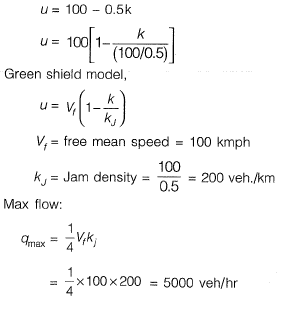Question 2. The uniform arrival and uniform service rates observed on an approach road to a signalized intersection are 20 and 50 vehicles/minute, respectively. For this signal, the red time is 30 s, the effective green time is 30 s, and the cycle length is 60 s. Assuming that initially there are no vehicles in the queue, the average delay per vehicle using the approach road during a cycle length (in s, round off to 2 decimal places) is______ .    [2019 : 2 Marks, Set-II]
Solution:
Arrival rate = 20 veh/min
Service rate = 50 veh/min
R= 30 sec, G1 = 30 sec, Cycle length = 60sec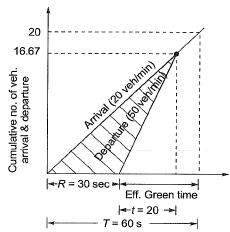Time corresponding to which no. of arrival becomes same as no. of departure.
=> 20 x (R + t) =50t
20 x (30 + t) =50t
600 + 20t =50t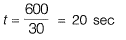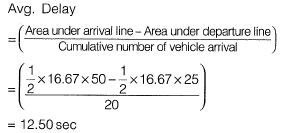Question 3. A vehicle is moving on a road of grade +4% at a speed of 20 m/s. Consider the coefficient of rolling friction as 0.46 and acceleration due to gravity as 10 m/s2. On applying brakes to reach a speed of 10 m/s, the required braking distance (in m, round off to nearest integer) along the horizontal, is     [2019 : 1 Mark, Set-II]
Solution: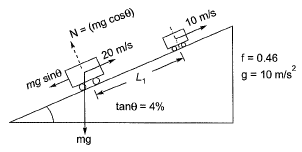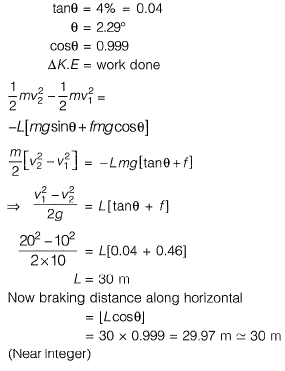Question 4.  The speed-density relationship in a mid-block section of a highway follows the Greenshield's model. If the free flow speed is vf and the jam density is kj, the maximum flow observed on this section is
(a)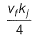(b)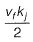(c)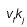(d)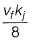(a)
Solution: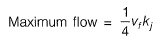Question 5. Traffic on a highway is moving at a rate of 360 vehicles per hour at a location. If the number of vehicles arriving on this highway follows Poisson distribution, the probability (round off to 2 decimal places) that the headway between successive vehicles lies between 6 and 10 seconds is_______.    [2019 : 2 Marks, Set-I]
Solution: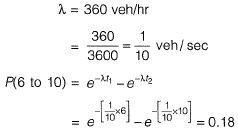Question 6.  Average free flow speed and the jam density observed on a road stretch are 60 km/h and 120 vehicles/km, respectively. For a linear speed- density relationship, the maximum flow on the road stretch (in vehicles/h) is_______ ,     [2019 : 2 Marks, Set-I]
Solution: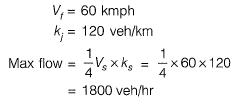Question 7. The maximum number of vehicles observed in any five minute period during the peak hour is 160. If the total flow in the peak hour is 1000 vehicles, the five minute peak hour factor (round off to 2 decimal places) is_______ .    [2019 : 1 Mark, Set-I]
Solution: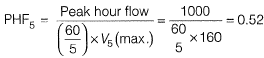Question 8. The space mean speed (kmph) and density (vehicles/km) of a traffic stream are linearly related. The free flow speed and jam density are 80 kmph and 100 vehicles/km respectively. The traffic flow (in vehicles/h, up to one decimal place) corresponding to a speed of 40 kmph is  _________    [2018 : 2 Marks, Set-II]
Solution:
Vf - Free mean speed = 50 kmph
kj = Jam density = 100 veh/km
As per linear model (green-shield)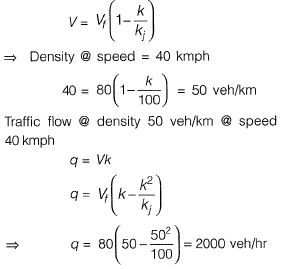Question 9. Peak Hour Factor (PHF) is used to represent the proportion of peak sub-hourly traffic flow within the peak hour. If 15-minute sub-hours are considered, the theoretically possible range of PHF will be     [2018 : 1 Mark, Set-II ]
(a) 0 to 1.0
(b) 0.25 to 0.75
(c) 0.25 to 1.0
(d) 0.5 to 1.0
Solution: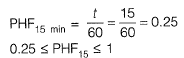Question 10. A well-designed signalized intersection is one in which the
(a) crossing conflicts are increased
(b) total delay is minimized
(c) cycle time is equal to the sum of red and green times in all phases
(d) cycle time is equal to the sum of red and yellow times in all phases     [2018 : 1 Mark, Set-I]

Question 11. The speed-density relationship for a road section is shown in the figure    [2018 : 1 Mark, Set-I]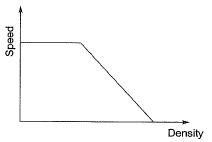The shape of the flow-density relationship is
(a) piecewise linear
(b) parabolic
(c) initially linear then parabolic
(d) initially parabolic then linear
Solution: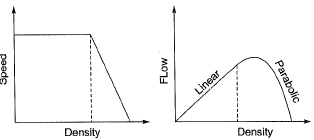q = vk
For initial values of k, v is constant
∴ q will very linearly with k
Later on, v varies linearly with k.
∴ q will vary parabolically with k.

Question 12. Two cars P and Q are moving in a racing track continuously for two hours. Assume that no other vehicles are using the track during this time. The expressions relating the distance travelled d (in km) and time t (in hours) for both the vehicles are given as
P : d=60t
Q : d = 60t2
Within the first one hour, the maximum space headway would be    [2017 : 2 Marks, Set-II]
(a) 15 km at 30 minutes
(b) 15 km at 15 minutes
(c) 30 km at 30 minutes
(d) 30 km at 15 minutes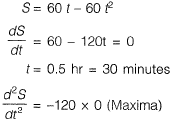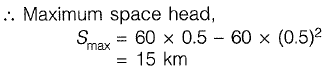Question 13. The safety within a roundabout and the efficiency of a roundabout can be increased, respectively by    [2017 : 2 Marks, Set-II]
(c)

Question 14. The queue length (in number of vehicles) versus time (in seconds) plot for an approach to a signalized intersection with the cycle length of 96 seconds is shown in the figure (not drawn to scale).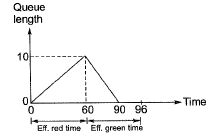At time, t = 0, the light has just turned red. The effective green time is 36 seconds, during which vehicles discharge at the saturation flow rate, s (in vph). Vehicles arrive at a uniform rate, v(in vph), throughout the cycle. Which one of the following statements is TRUE?    [2017 : 2 Marks, Set-I]
(a) v = 600 vph, and for this cycle, the average stopped delay per vehicle = 30 seconds.
(b) s = 1800 vph, and for this cycle, the average stopped delay per vehicle = 28.125 seconds.
(c) v = 600 vph, and for this cycle, the average stopped delay per vehicle = 45 seconds.
(d) s = 1200 vph, and for this cycle, the average stopped delay per vehicle = 28.125 seconds.
(b)
Solution: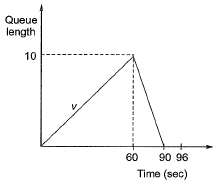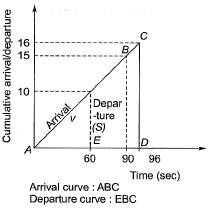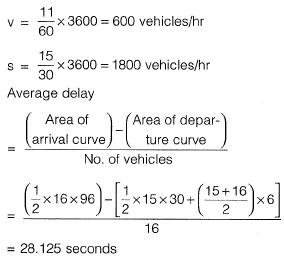Question 15. Vehicles arriving at an intersection from one of the approach roads follow the Poisson distribution. The mean rate of arrival is 900 vehicles per hour. If a gap is defined as the time difference between two successive vehicle arrivals (with vehicles assumed to be points), the probability (up to four decimal places) that the gap is greater than 8 seconds is __________.     [2017 : 1 Mark, Set-I]
Solution:

λ = 900 veh/hour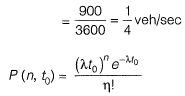Probability that time gap (time headway) betwen successive vehicles is greater than 8 seconds,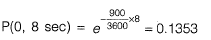Offer running on EduRev: Apply code STAYHOME200 to get INR 200 off on our premium plan EduRev Infinity!

,

,

,

,

,

,

,

,

,

,

,

,

,

,

,

,

,

,

,

,

,

;